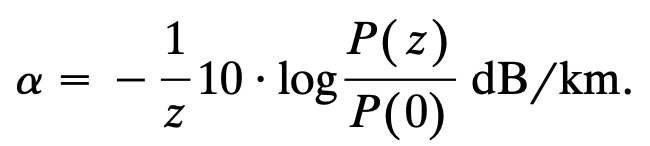# Optical photons absorption and diffusion coefficients

Dear @blefebvre

Thanks for your illustrated explanation. This helps a lot.
Here’s my understanding of the absorption / diffusion coefficient. Please see if i’m right.

1. The total signal loss of fiber is defined as : `Total Loss = Absorption + Diffusion`.
And this attenuation factor (total loss) of fiber is given by the fomular :`z` is the length of fiber,` P(0)`, `P(z)` is the optical intensity for corresponding position of fiber.

2. From the brochure or papers, absoption / diffusion coefficients could be obtained. From the unit `dB/km`, we could conclude that the coefficients are defined by the above formula.

Here are my questions:

1. Could you please explain what the 10cm in the last post means? What is the calculatio formular of attenuation coefficient? Seems absorption coefficient is the inverse of absorption length?
2. In the following post, the diffusion is defined as the inverse of mean free length.
`. https://fluka-forum.web.cern.ch/t/diffusion-coefficient/1761/3`
So the `Diffusion` in `OPT-PROP` card this is not the common definition of diffusion coefficient in papers.

Dear @songhg19,

The absorption coefficient is the inverse of the absorption length in units of cm^{-1}. The intensity of a beam of optical photon is reduced by a factor exp(-1) after traversing one absorption length of an optical material.

Similarly, the diffusion coefficient is the inverse of the diffusion length. A fraction exp(-1) of a beam of optical photons remains unscattered after traversing one diffusion length.

The absorption coefficient defined in FLUKA corresponds to your alpha parameter the only difference being that by using units of decibels you assume the intensities goes as 10^{-alpha/10×z} while FLUKA assumes the intensity goes as exp(-Cz). Evidently both approaches are physically equivalent. The two coefficients are related by C=0.1×ln(10)×alpha (an extra conversion from cm to km could be necessary).

Note that in FLUKA, dispersion processes do not contribute to a “loss” in intensity because optical photons are merely scattered, not killed. For the special case of an optical fiber, diffusion processes could indeed indirectly contribute to the total loss in FLUKA, but this depends on the specifics of your simulation which I am not in a position to comment on this forum.

Dear @blefebvre